# Deep Learning Libraries Giuseppe Attardi General DL Libraries

• Slides: 18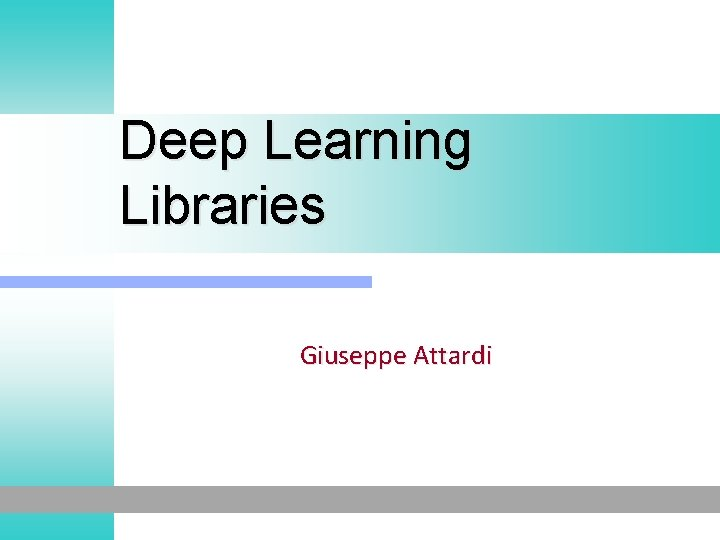Deep Learning Libraries Giuseppe Attardi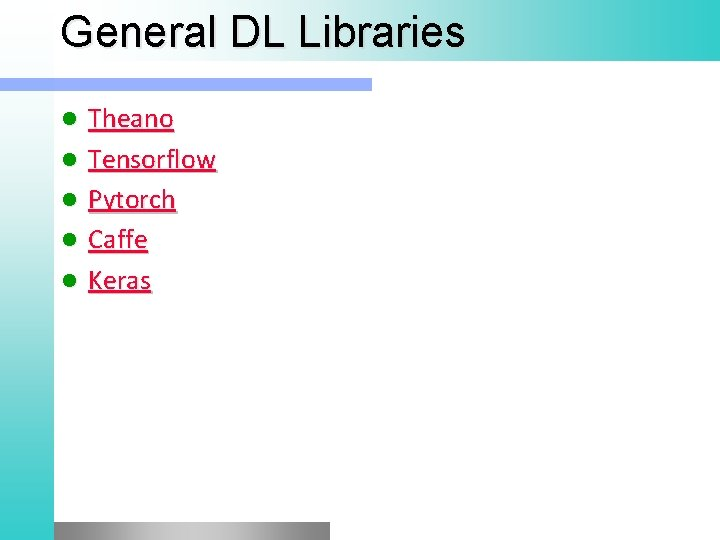General DL Libraries l l l Theano Tensorflow Pytorch Caffe KerasCaffe l l Deep Learning framework from Berkeley focus on vision coding in C++ or Python Network consists in layers: layer = { name = “data”, …} layer = { name = “conv”, . . . } l data and derivatives flow through net as blobs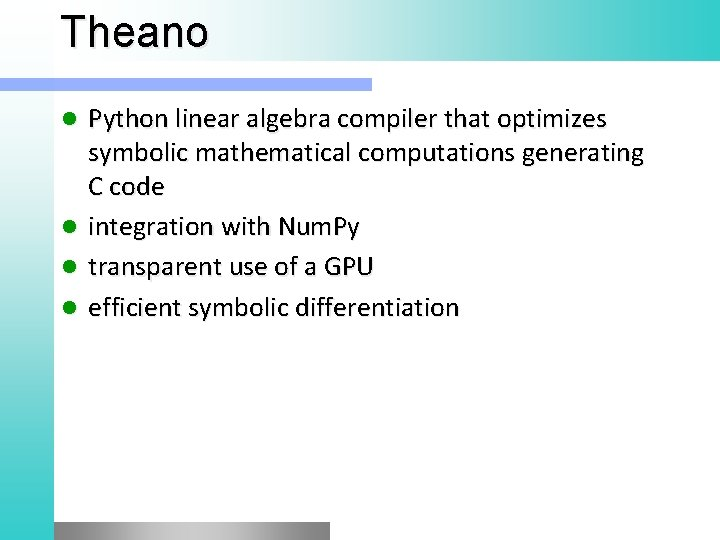Theano l l Python linear algebra compiler that optimizes symbolic mathematical computations generating C code integration with Num. Py transparent use of a GPU efficient symbolic differentiation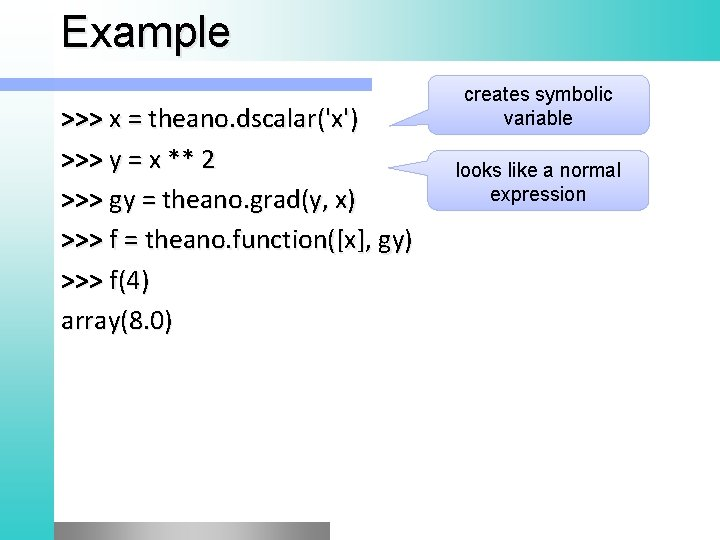Example >>> x = theano. dscalar('x') >>> y = x ** 2 >>> gy = theano. grad(y, x) >>> f = theano. function([x], gy) >>> f(4) array(8. 0) creates symbolic variable looks like a normal expression2 -layer Neural Network z 1 = X. dot(W 1) + b 1 # first layer a 1 = tanh(z 1) # activation z 2 = a 1. dot(W 2) + b 2 # second layer y_hat = softmax(z 2) # output probabilities # class prediction = T. argmax(y_hat, axis=1) # the loss function to optimize loss = categorical_crossentropy(y_hat, y). mean()Gradient Descent d. W 2 = T. grad(loss, W 2) db 2 = T. grad(loss, b 2) d. W 1 = T. grad(loss, W 1) db 1 = T. grad(loss, b 1) gradient_step = theano. function([X, y], updates = ((W 2, W 2 – learn_rate * d. W 2), (W 1, W 1 – learn_rate* d. W 1), (b 2, b 2 – learn_rate* db 2), (b 1, b 1 – learn_rate* db 1)))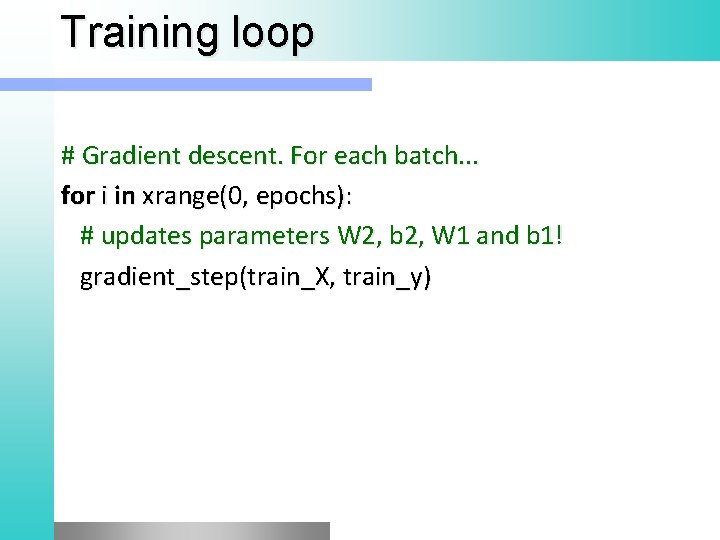Training loop # Gradient descent. For each batch. . . for i in xrange(0, epochs): # updates parameters W 2, b 2, W 1 and b 1! gradient_step(train_X, train_y)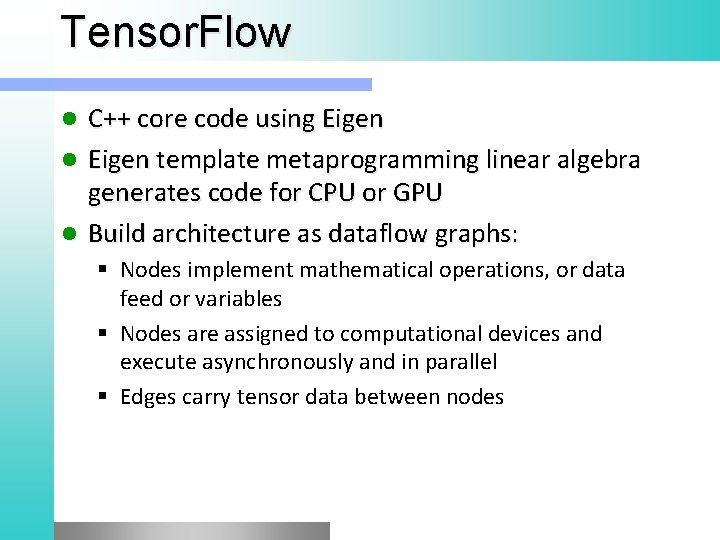Tensor. Flow C++ core code using Eigen l Eigen template metaprogramming linear algebra generates code for CPU or GPU l Build architecture as dataflow graphs: l § Nodes implement mathematical operations, or data feed or variables § Nodes are assigned to computational devices and execute asynchronously and in parallel § Edges carry tensor data between nodesTraining Gradient based machine learning algorithms l Automatic differentiation l Define the computational architecture, combine that with your objective function, provide data -- Tensor. Flow handles computing the derivatives lPerformance Support for threads, queues, and asynchronous computation l Tensor. Flow allows assigning graph to different devices (CPU or GPU) lKerasKeras is a high-level neural networks API, written in Python and capable of running on top of Tensor. Flow, CNTK, or Theano. l Guiding principles l § User friendlyness, modularity, minimalism, extensibility, and Python-nativeness. l Keras has out-of-the-box implementations of common network structures: § convolutional neural network § Recurrent neural network l Runs seamlessly on CPU and GPU.Outline l Install Keras § Import libraries § Load image data § § § Preprocess input Define model Compile model Fit model on training data Evaluate modelInstall Keras Full instructions l Basic instructions: l § Install backend: Tensorflow or Theano > pip install keras30 sec. example from keras. models import Sequential from keras. layers import Dense model = Sequential() model. add(Dense(units=64, activation='relu', input_dim=100)) input_dim=100 model. add(Dense(units=10, activation='softmax')) model. compile(loss='categorical_crossentropy', optimizer='sgd', metrics=['accuracy']) optimizer model. compile(loss=keras. losses. categorical_crossentr opy, optimizer=keras. optimizers. SGD(lr=0. 01, momentum=0. 9, nesterov=True)) model. fit(x_train, y_train, epochs=5, batch_size=32 ) classes = model. predict(x_test, batch_size=128)Keras Tutorial l Run tutorial at: § http: //attardi 4. di. unipi. it: 8000/user/attardi/notebooks/MNIST%20 in%20 Keras. ipynbDeep. NL l l Deep Learning library for NLP C++ with Eigen Wrapper for Python, Java, PHP automatically generated with SWIG Git repository